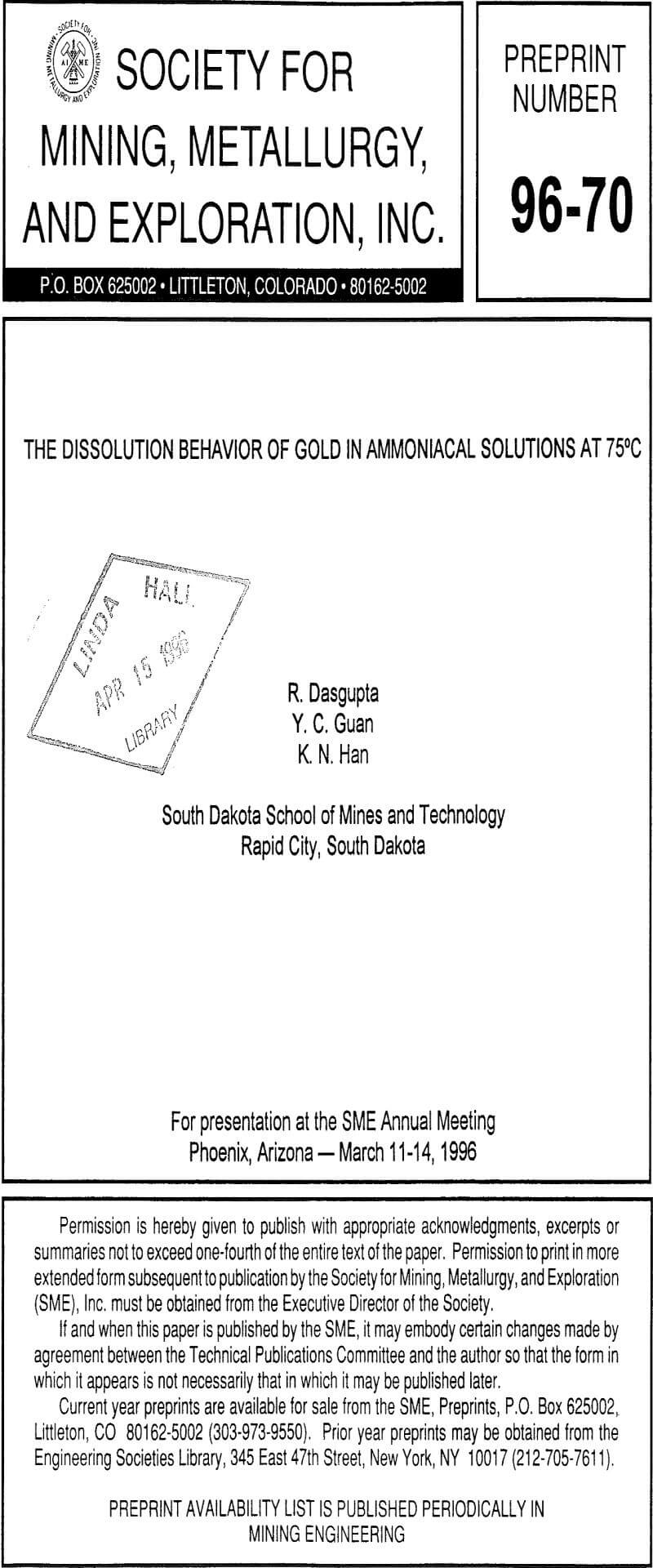# Effect of Cupric Ammine on Gold Dissolution Rate

The dissolution of gold in ammoniacal solutions cannot take place in the absence of oxidants. Cupric ammine can act as an oxidant in this system according to Equation 3.

The effect of cupric ammine concentration on the dissolution rate of gold was investigated in a solution of 0.6 M total ammonia concentration (0.1 M (NH4)2SO4 and 0.4 M NH3). The cupric ammine solution was prepared by adding known concentrations of copper sulfate (CuSO4.5H2O) and free ammonia in the ratio of 1:4. Figure 2 shows the Tafel polarization curves for the dissolution of gold at different cupric ammine concentrations. It can be observed from these curves that the dissolution potential of the system increases with the increase of the concentration of cupric ammine. Therefore, cupric ammine can be successfully used as an oxidant for the dissolution of gold in ammoniacal solutions.It can also be seen from the curves in Figure 2 that variation in the cupric ammine concentration had no effect on the anodic portion of the curve. This suggests that cupric ammine has no effect on the anodic reaction which is given by Equation 1. Hence, it can be concluded that the dissolution rate of gold is increased in the presence of cupric ammine due to the increase in the cathodic reaction only.

From the extrapolation of the anodic and cathodic portions of the Tafel polarization curves, the dissolution current density was obtained. The rate of dissolution was calculated from the following relation:

Rate = i/nF……………………………………………………………………(8)

where,

i = dissolution current density (A/cm²)
F = Faraday constant, 96500 C/mole.
n = number of electrons transferred in the process = 1

Figure 3 shows the plots of log (Rate) versus log [Cu(NH3)4+²] for the electrochemical investigation. The order of the reaction found from the slope of the plot is 0.38.

## Effect of Total Ammonia on Gold Dissolution Rate

In ammoniacal solution, an equilibrium relationship exists between [NH3], [NH4+] and pH. If the variable total ammonia ([NH3] + [NH4+]) is taken into consideration, the ratio of [NH4+] to [NH3] will change with pH according to the equation (Vu and Han, 1986):

[NH3]/[NH4+] = 10pH-9.27…………………………………………………………..(9)

The above relationship shows that in the ammonia- water system, if the ratio of the [NH3] to [NH4+] is kept constant, the pH of the solution remains constant. Experiments were conducted in which the total ammonia concentration in the solution ([NH3]+[NH4+]) was varied from 0.1 M to 5.0 M but keeping the cupric ammine concentration constant at 0.002 M. The pH was kept constant by keeping the ratio of [NH3] to [NH4+] constant at 3.

The results of the electrochemical investigation showed that the dissolution potential decreases and the anodic current density increases with the increase of the total ammonia concentration. In the current investigation, the total ammonia concentration was increased which leads to an increase in the free ammonia concentration. Consequently, the equilibrium potential of the anodic reaction decreases. A decrease of the equilibrium potential of the anodic reaction leads to a decrease of the overall dissolution potential of the mixed electrode system. This explains the observed decrease of the dissolution potential with the increase in the total ammonia concentration. From the anodic reaction (Equation 1), it is also evident that an increase of free ammonia concentration will drive the reaction forward and consequently increase the anodic current density. This explains the observations noted from the experimental curves.

It was also noted that the cathodic current density decreases as the concentration of total ammonia was increased. Since free ammonia is a product of the cathodic reaction, an increase of free ammonia concentration drives the cathodic reaction backward and decreases the cathodic current density. Figure 4 shows the dependence of the dissolution rates (calculated from the dissolution currents by extrapolation of the Tafel plots) with the concentration of total ammonia in the system. The plot shows that the overall dissolution rate is independent of the total ammonia concentration. This observation can be explained by the fact that the positive and negative effects of the total ammonia concentration on the anodic and cathodic portions of the Tafel curves neutralize each other and hence maintain the overall dissolution rate constant.